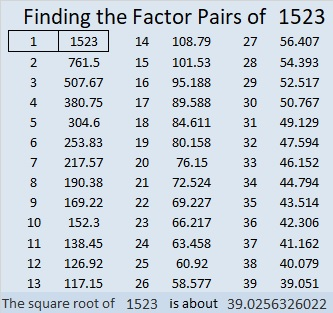# 1523 Mystery Puzzle

### Today’s Puzzle:

My newly turned 10-year-old granddaughter likes making Find the Factors 1 -12 Puzzles with me. Unfortunately, at the beginning of the month, she was in an accident. Her 12-year-old cousin hadn’t ever made a puzzle before but helped me make this one to wish her a speedy recovery. (Thankfully, she is almost fully recovered now.)### Factors of 1523:

• 1523 is a prime number.
• Prime factorization: 1523 is prime.
• 1523 has no exponents greater than 1 in its prime factorization, so √1523 cannot be simplified.
• The exponent in the prime factorization is 1. Adding one to that exponent we get (1 + 1) = 2. Therefore 1523 has exactly 2 factors.
• The factors of 1523 are outlined with their factor pair partners in the graphic below.How do we know that 1523 is a prime number? If 1523 were not a prime number, then it would be divisible by at least one prime number less than or equal to √1523. Since 1523 cannot be divided evenly by 2, 3, 5, 7, 11, 13, 17, 19, 23, 29, 31, or 37, we know that 1523 is a prime number.

### Another Fact about the Number 1523:

1523 is the difference of two consecutive squares:
762² – 761² = 1523.